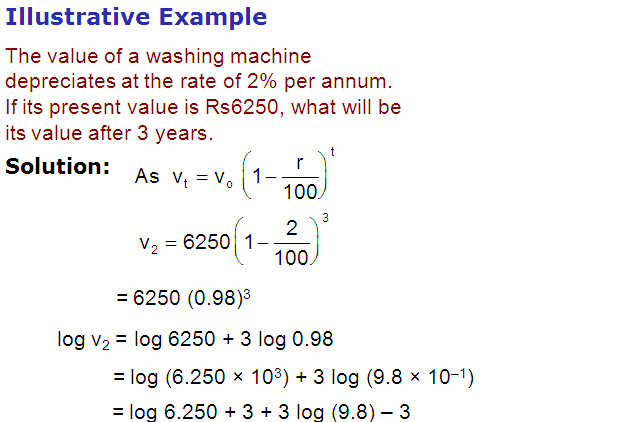# SCC Education

## Fundamental laws of logarithms with solved example part 2

In mathematics, the logarithm is the inverse

operation to exponentiation. That means

thelogarithm of a number is the exponent to

which another fixed value, the base, must be

raised to produce that number. In simple cases

the logarithm counts repeated multiplication

log ,log table